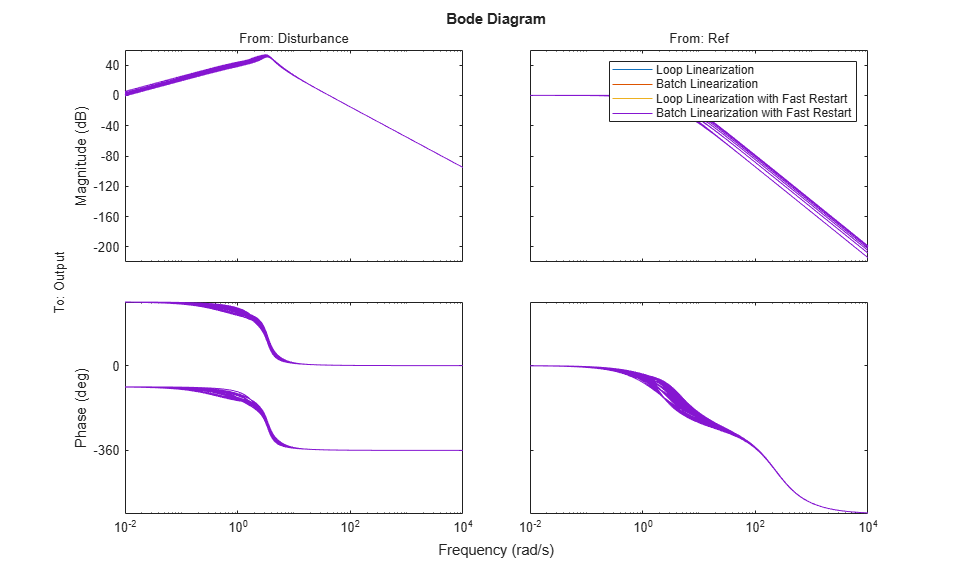# Improve Linear Analysis Performance

This example shows how to use the `fastRestartForLinearAnalysis` function to speed up multiple calls to compiling functions in Simulink® Control Design™ software such as `findop` and `linearize`.

### Run Linear Analysis Commands in a Loop

In this example, you trim and linearize a closed-loop engine speed control model. You vary the PI control parameters and observe how the closed-loop behavior changes at steady state. Since `linearize` and `findop` are called in a loop, the model compiles 2*N + 1 times including the first call to `operspec`.

Open the engine speed control model and obtain the linear analysis points from the model.

```mdl = 'scdspeedctrl'; open_system(mdl) io = getlinio(mdl); fopt = findopOptions('DisplayReport','off'); ```Configure the PI controller to use the base workspace variables `kp` and `ki`.

```blk = [mdl,'/PID Controller']; set_param(blk,'P','kp'); set_param(blk,'I','ki'); ```

Create a grid of parameter values to vary.

```vp = 0.0005:0.0005:0.003; vi = 0.0025:0.0005:0.005; [KP,KI] = ndgrid(vp,vi); N = numel(KP); sz = size(KP); ```

Initialize the base workspace variables.

```kp = KP(1); ki = KI(1); ```

Run the loop and record execution time.

```t = cputime; ops = operspec(mdl); for i = N:-1:1 kp = KP(i); ki = KI(i); % Trim the model. op = findop(mdl,ops,fopt); [j,k] = ind2sub(sz,i); % Linearize the model. sysLoop(:,:,j,k) = linearize(mdl,io,op); end ```

Calculate the elapsed time.

```timeElapsedLoop = cputime - t; ```

### Run Linear Analysis Commands in Batch

Rather than loop over the parameters, `findop` and `linearize` can accept a batch parameter variation structure directly to reduce the number of times the model compiles. In this case, the model compiles three times with calls to `operspec`, `findop`, and `linearize`.

Run and record execution time.

```t = cputime; ops = operspec(mdl); ```

Create the batch parameter structure.

```params(1).Name = 'kp'; params(1).Value = KP ; params(2).Name = 'ki'; params(2).Value = KI ; ```

Trim the model across the parameter set.

```op = findop(mdl,ops,params,fopt); ```

Linearize the model across the parameter and operating point set.

```sysBatch = linearize(mdl,io,op,params); ```

Calculate the elapsed time.

```timeElapsedBatch = cputime - t; ```

### Run Linear Analysis Functions in Loop with Fast Restart

The `fastRestartForLinearAnalysis` function configures the model to minimize compilations even when compiling functions are run inside a loop. The model compiles with calls to `operspec`, `findop`, and `linearize` in a loop.

Run the loop and record execution time with fast restart for linear analysis enabled.

```t = cputime; ```

Enable fast restart for linear analysis. Provide linear analysis points to minimize compilations between calls to `findop` and `linearize`.

```fastRestartForLinearAnalysis(mdl,'on','AnalysisPoints',io); ```

Trim and linearize the model in a loop.

```ops = operspec(mdl); for i = N:-1:1 kp = KP(i); ki = KI(i); % Trim the model. op = findop(mdl,ops,fopt); [j,k] = ind2sub(sz,i); % Linearize the model. sysFastRestartLoop(:,:,j,k) = linearize(mdl,io,op); end ```

Turn off fast restart for linear analysis, which uncompiles the model.

```fastRestartForLinearAnalysis(mdl,'off'); ```

Calculate the elapsed time.

```timeElapsedFastRestartLoop = cputime - t; ```

### Run Linear Analysis Functions in Batch with Fast Restart

You can further improve performance by enabling fast restart for linear analysis and executing the batch `linearize` and `findop` functions. In this case, the model compiles once with calls to `operspec`, `findop`, and `linearize`.

Run and record execution time with fast restart for linear analysis on.

```t = cputime; ```

Enable fast restart for linear analysis. Provide linear analysis points to minimize compilations between calls to `findop` and `linearize`.

```fastRestartForLinearAnalysis(mdl,'on','AnalysisPoints',io) ```

Create the batch parameter structure.

```params(1).Name = 'kp'; params(1).Value = KP ; params(2).Name = 'ki'; params(2).Value = KI ; ```

Trim the model across the parameter set.

```ops = operspec(mdl); op = findop(mdl,ops,params,fopt); ```

Linearize the model across the parameter and operating point set.

```sysFastRestartBatch = linearize(mdl,io,op,params); ```

Disable fast restart for linear analysis, which uncompiles the model.

```fastRestartForLinearAnalysis(mdl,'off'); ```

Calculate the elapsed time.

```timeElapsedFastRestartBatch = cputime - t; ```

### Compare the Results

Compare the linearization results of the four methods. The bode plot below shows that each method returns the same results.

```compareIdx = 1:N; bode(... sysLoop(:,:,compareIdx), ... sysBatch(:,:,compareIdx), ... sysFastRestartLoop(:,:,compareIdx), ... sysFastRestartBatch(:,:,compareIdx)); legend(... 'Loop Linearization', ... 'Batch Linearization', ... 'Loop Linearization with Fast Restart', ... 'Batch Linearization with Fast Restart') ```Compile the elapsed time and speed-up ratio for each method in a table. You can obtain significant performance gains using batch trimming and linearization as well as `fastRestartForLinearAnalysis`.

```Method = ["Loop","Batch","Fast Restart Loop","Fast Restart Batch"]'; TimeElapsed = [timeElapsedLoop timeElapsedBatch ... timeElapsedFastRestartLoop timeElapsedFastRestartBatch]'; SpeedUpFactor = TimeElapsed(1)./TimeElapsed; TimeElapsedTable = table(Method,TimeElapsed,SpeedUpFactor) ```
```TimeElapsedTable = 4x3 table Method TimeElapsed SpeedUpFactor ____________________ ___________ _____________ "Loop" 50.92 1 "Batch" 12.58 4.0477 "Fast Restart Loop" 15.26 3.3368 "Fast Restart Batch" 12.91 3.9442 ```

```bdclose(mdl) ```